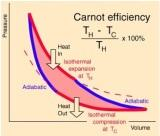## April 5, 2012

### The Carnot's Cycle

Introduction

The Carnot's cycle was proposed by Sadi Carnot (1796-1832), who is considered to be the founder of thermodynamics. The cycle describes a sequence of steps that can be performed only in an ideal engine, which transforms heat into work. The Cycle was a culmination of the research done by Carnot in thermodynamics. By the end of the eighteenth century, many engineers were doing experiments on engines used to perform especialized work, using heat as the source of energy. For example, many engines were designed using as source or energy the heat produced by combustion of coal or wood.
A common goal of all those engineers were to create machines that could be as effcient as possible in using the heat provided. Based on the study of these machines, Carnot discovered the cycle as an ideal model, and demonstrated that this could not be improved.
Phases of the Carnot's Cycle

A diagram of the Carnot's Cycle is shown in the figure down there. The sequence of steps in the Carnot's
cycle are the following:
² Adiabatic compression from temperature T1 to T2, where T2 < T1;
² Isothermal expansion at the temperature T1
² Adiabatic expansion to T2
² Isothermal compression
We describe each of these phases of the cycle next.The first phase of Carnot's cycle consists of an adiabatic compression of the material in the engine. An adiabatic transformation is one in which no heat is gained or lost. In this kind of process, the energy is conserved inside the boundaries of the system. This condition can be described in mathematical therms as dQ = 0;
where Q is the variable representing the amount of heat in the system.
However, according to the first law of thermodynamics,
dE = dQ-dW;
i.e., the variation of internal energy is equal to the variation of temperature minus the
variation of work done by the system. Therefore
dE = -dW = -pdV;
where V is the volume of the system. Thus, to have an adiabatic process we must have change in volume of the system.

A diagram of the Carnot's Cycle, with a measure of its eficiency.

Acordingly, the first phase of the Carnot's Cycle is defined by a change in volume of the system. This change is made in such a way that energy is transformed from heat into movement, and that will result in the change of volume. The temperature in the system, consequently, changes from T1 to T2.
However, the process of changing heat into work cannot be done indefinitely, due to restrictions from the 2nd law of thermodynamics. This implies that the system will at some point loose e±ciency, and heat energy cannot be further transformed into work.

### Isothermal expansion/compression

These two phase are complementary to the phases described above. An isothermal process is one in which the temperature remains constant. To have expansion, or compression at isothermal conditions, some energy must exchanged with other systems, and therefore work can be done.
In the second phase of the Carnot's cycle, the system will expand at a the same temperature. This will promote the transformation of energy from heat to a mechanical form. It is important to mention that this process is not reversible, and it is there that the limitation is, acording to the 2nd law of thermodynamics.
The last phase of the Carnot's cycle is similar, but here a contraction is done, instead of an expansion. This makes the system go back to the same state it was at the beginning of the cycle, and a new cycle can start.

### Importance of the Carnot's Cycle

The Carnot's cycle is important since it describes an optimal system, where heat energy is used at its best. However, it has consequences beyond the simple study of engines. It can be also used to describe theoretical limits of the transformation of heat into physical work. In this sense it is applicable not only to mechanical models, but also to other kinds of energy.
One of the consequences of the second law of thermodynamics is that heat cannot be transformed completely in work. Heat is a type of energy that has high entropy. Acording to the 2nd law of thermodynamics, entropy in a system can only increase with time. Thus, this gives a limit on the amount of energy that can be tranfered from heat to work.
Carnot's cycle is a physical expression of the consequence of the 2nd law of thermodynamics for engines and other related physical systems. It quanti¯es the loss of energy that must occur whenever we try to convert heat into other types of physical energy.